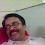# Excel: 4 Ways to Sum Digits in a Number

Assuming a value is entered in cell B4 i.e. 45454. The sum of the digits of this number is 22.

## 1. SUM & INDEX Formula

=SUM(INDEX(1*(MID(B4,ROW(INDIRECT("1:"&LEN(B4))),1)),,))

Here is a breakdown of the formula:

• `MID(B4,ROW(INDIRECT("1:"&LEN(B4))),1)` extracts each digit from the cell B4. It uses the MID function to take one character at a time, starting from the first character (1), up to the length of the number (LEN(B4)).
• `1*(MID(B4,ROW(INDIRECT("1:"&LEN(B4))),1))` converts the extracted digits from text to numeric values by multiplying them by 1.
• `INDEX(...)` is used to create an array of the individual digits.
• `SUM(...)` calculates the sum of all the digits in the array.

## 2. SUMPRODUCT & INDIRECT Formula

=SUMPRODUCT(MID(B4,ROW(INDIRECT("1:" & LEN(B4))),1)*1)

Instead of using SUM and INDEX, we are using the SUMPRODUCT(...) which multiplies each digit by 1 and then sums up all the products, resulting in the sum of the digits.

## 3. SUMPRODUCT & OFFSET Formula

=SUMPRODUCT(MID(B4,ROW(OFFSET(\$A\$1,,,LEN(B4))),1)+0)

The ROW and OFFSET functions are used to generate a dynamic array of row numbers corresponding to the length of the number. +0 is added to convert the extracted digits from text to numeric values.

## 4. Array Formula

Those who don't mind hitting Ctrl Shift Enter instead of regular ENTER button. The array formula is generally considered complex and difficult to handle and maintain.

=SUM(MID(B4,ROW(INDIRECT("1:" & LEN(B4))),1)*1)
Note : Press F2 and Hit Ctrl Shift Enter to confirm the above array formula.
Related Posts
ShareDeepanshu founded ListenData with a simple objective - Make analytics easy to understand and follow. He has over 10 years of experience in data science. During his tenure, he worked with global clients in various domains like Banking, Insurance, Private Equity, Telecom and HR.

3 Responses to "Excel: 4 Ways to Sum Digits in a Number"
1.plz explain it

2.There is one more.
ctrl+shift+enter:
=SUM(IFERROR(1*MID(B4,ROW(\$1:\$99),1),))

3.THE FORMULAS WONT WORK FOR CELL WITH LINE BREAKS NUMBER VALUE

Next → ← Prev
Looks like you are using an ad blocker!

To continue reading you need to turnoff adblocker and refresh the page. We rely on advertising to help fund our site. Please whitelist us if you enjoy our content.